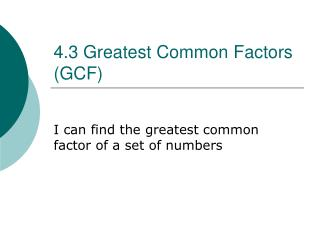DownloadDownload Presentation4.3 Greatest Common Factors (GCF)

# 4.3 Greatest Common Factors (GCF)

Download Presentation## 4.3 Greatest Common Factors (GCF)

- - - - - - - - - - - - - - - - - - - - - - - - - - - E N D - - - - - - - - - - - - - - - - - - - - - - - - - - -
##### Presentation Transcript

1. 4.3 Greatest Common Factors (GCF) I can find the greatest common factor of a set of numbers

2. Review A factor is number that is multiplied by another number to get a product

3. The greatest common factor is the largest factor that two or more numbers share. Factors of 24: Factors of 36: Common factors: 1, 2, 3, 4, 6, 8, 12, 24 1, 2, 3, 4, 6, 9, 12, 18, 36 1, 2, 3, 4, 6, 12 The greatest common factor (GCF) of 24 and 36 is 12. Example 1 shows three different methods for finding the GCF.

4. Additional Example 1A: Finding the GCF Find the GCF of the set of numbers. 28 and 42 Method 1: List the factors. factors of 28: factors of 42: List all the factors. 1, 2, 4, 7, 14, 28 1, 2, 3, 6, 7, 14, 21, 42 Circle the GCF. The GCF of 28 and 42 is 14.

5. Check It Out: Example 1A Find the GCF of the set of numbers. 18 and 36 Method 1: List the factors. factors of 18: factors of 36: List all the factors. 1, 2, 3, 6, 9, 18 1, 2, 3, 4, 6, 9, 12, 18, 36 Circle the GCF. The GCF of 18 and 36 is 18.

6. Try on your Own • 16 & 28 • 24 & 56

7. Guided: using Distributive Property • 15 + 25 • 36 + 72

8. Try on your own • 10 + 90= • 45 + 72=

9. LCM • LCM: Least Common Multiple: The smallest number, other than zero, that is a multiple of two or more given numbers

10. Guided Practice • 5 & 6 • 9 & 11

11. Try on Your own • 3 & 7 • 4 & 6